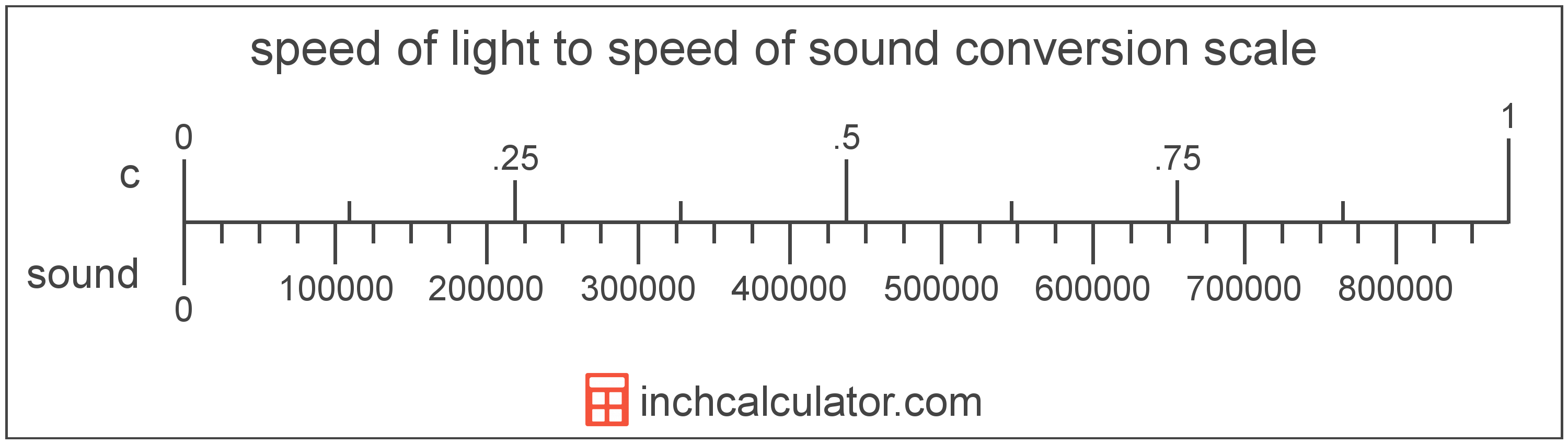# Speed of Sound to Speed of Light Converter

Enter the speed in speed of sound below to get the value converted to speed of light.

Results in Speed of Light:1 sound = 1.1441E-6 c
Hint: use a scientific notation calculator to convert E notation to decimal

Do you want to convert speed of light to speed of sound?

## How to Convert Speed of Sound to Speed of Light

To convert a measurement in speed of sound to a measurement in speed of light, multiply the speed by the following conversion ratio: 1.1441E-6 speed of light/speed of sound.

Since one speed of sound is equal to 1.1441E-6 speed of light, you can use this simple formula to convert:

speed of light = speed of sound × 1.1441E-6

The speed in speed of light is equal to the speed in speed of sound multiplied by 1.1441E-6.

For example, here's how to convert 500,000 speed of sound to speed of light using the formula above.
speed of light = (500,000 sound × 1.1441E-6) = 0.572062 cSpeed of sound and speed of light are both units used to measure speed. Keep reading to learn more about each unit of measure.

## What is the Speed of Sound?

The speed of sound is the distance a sound wave travels through an elastic medium. The speed of sound through air at 20 °C is equal to 343 meters per second, or roughly 767 miles per hour.

Speed of sound can be abbreviated as sound; for example, 1 speed of sound can be written as 1 sound.

## What is the Speed of Light?

The speed of light is equal to exactly 299,792,458 meters per second, or 670,616,629 miles per hour. The definition of the speed of light is actually derived from the most recent 1983 international definition of the meter.

Speed of light can be abbreviated as c; for example, 1 speed of light can be written as 1 c.

## Speed of Sound to Speed of Light Conversion Table

Table showing various speed of sound measurements converted to speed of light.
Speed Of Sound Speed Of Light
1 sound 0.0000011441 c
2 sound 0.0000022882 c
3 sound 0.0000034324 c
4 sound 0.0000045765 c
5 sound 0.0000057206 c
6 sound 0.0000068647 c
7 sound 0.0000080089 c
8 sound 0.000009153 c
9 sound 0.000010297 c
10 sound 0.000011441 c
100 sound 0.000114 c
1,000 sound 0.001144 c
10,000 sound 0.011441 c
100,000 sound 0.114412 c
1,000,000 sound 1.1441 c

## References

1. CK-12, Speed of Sound, https://www.ck12.org/physics/speed-of-sound/lesson/Speed-of-Sound-MS-PS/
2. National Institute of Standards and Technology, SI Redefinition: Meter, https://www.nist.gov/si-redefinition/meter